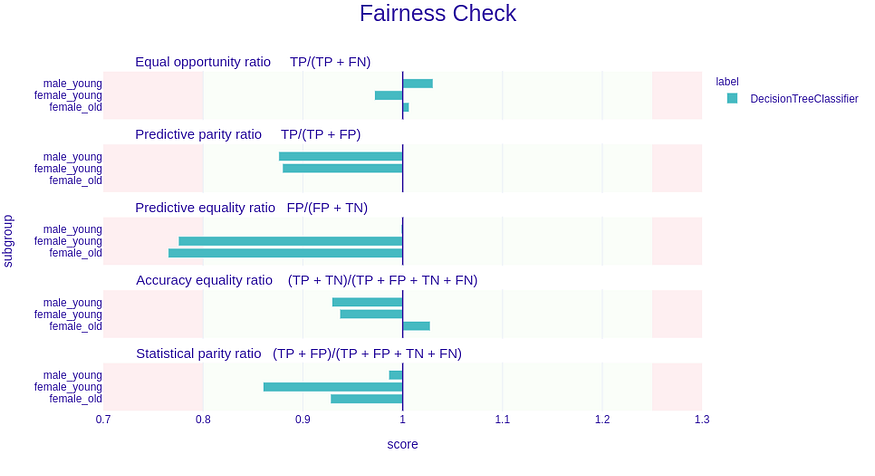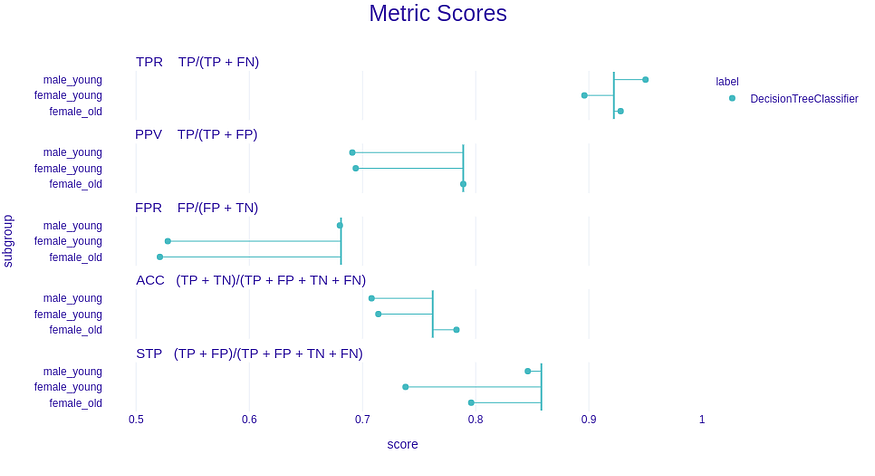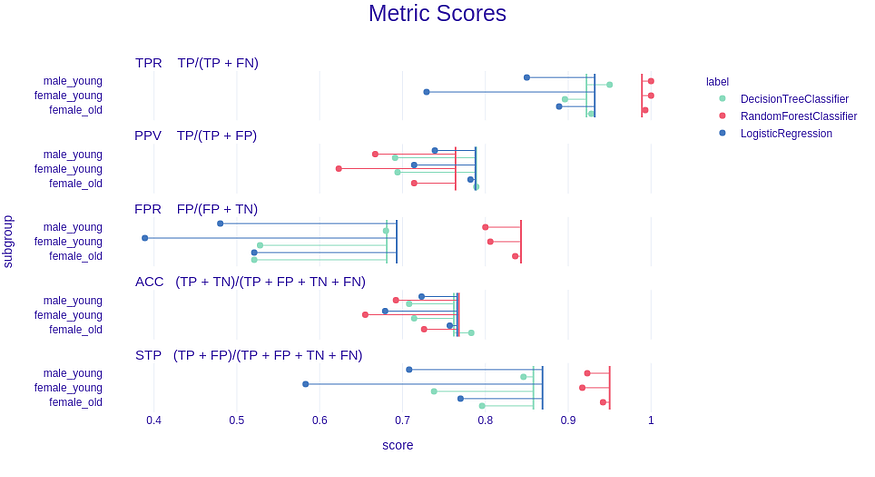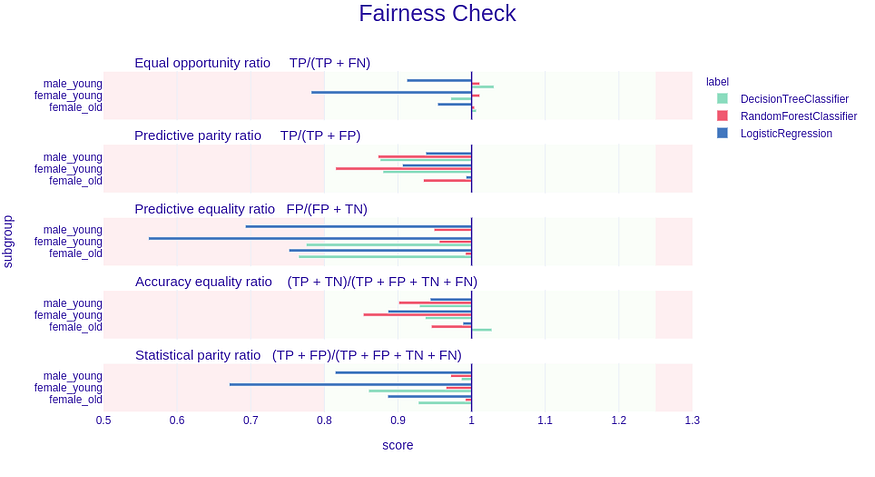# How to easily check if your Machine Learning model is fair?

Machine learning models deployed today -- as will many more in the future -- impact people and society directly. With that power and influence resting in the hands of Data Scientists and machine learning engineers, taking the time to evaluate and understand if model results are fair will become the linchpin for the future success of AI/ML solutions. These are critical considerations, and using a recently developed fairness module in the dalex Python package is a unified and accessible way to ensure your models remain fair.

By Jakub Wiśniewski, data science student and research software engineer in MI2 DataLab.Photo by Eric Krull on Unsplash.

We live in a world that is getting more divided each day. In some parts of the world, the differences and inequalities between races, ethnicities, and sometimes sexes are aggravating. The data we use for modeling is, in the major part, a reflection of the world it derives from. And the world can be biased, so data and therefore the model will likely reflect that. We propose a way in which ML engineers can easily check if their model is biased. Our fairness tool now works only with classification models.

### Case study

To showcase the abilities of the dalex fairness module, we will be using the well-known German Credit Data dataset to assign risk for each credit-seeker. This simple task may require using an interpretable decision tree classifier.

Once we have dx.Explainer we need to execute the method model_fairness(), so it can calculate all necessary metrics among the subgroups from the protected vector, which is an array or a list with sensitive attributes denoting sex, race, nationality, etc., for each observation (individual). Apart from that, we will need to point which subgroup (so which unique element of protected) is the most privileged, and it can be done through privileged parameter, which in our case will be older males.

This object has many attributes, and we will not go through each and every one of them. A more detailed overview can be found in this tutorial. Instead, we will focus on one method and two plots.

### So is our model biased or not?

This question is simple, but because of the nature of bias, the response will be: it depends. But this method measuring bias from different perspectives so that no bias model can go through. To check fairness, one has to use fairness_check() method.

```fobject.fairness_check(epsilon = 0.8) # default epsilon

```

The following chunk is the console output from the code above.

```Bias detected in 1 metric: FPR

Conclusion: your model cannot be called fair because 1 metric score exceeded acceptable limits set by epsilon.
It does not mean that your model is unfair but it cannot be automatically approved based on these metrics.

Ratios of metrics, based on 'male_old'. Parameter 'epsilon' was set to 0.8 and therefore metrics should be within (0.8, 1.25)
TPR       ACC       PPV       FPR       STP
female_old    1.006508  1.027559  1.000000  0.765051  0.927739
female_young  0.971800  0.937008  0.879594  0.775330  0.860140
male_young    1.030369  0.929134  0.875792  0.998532  0.986014

```

The bias was spotted in metric FPR, which is the False Positive Rate. The output above suggests that the model cannot be automatically approved (like said in the output above). So it is up to the user to decide. In my opinion, it is not a fair model. Lower FPR means that the privileged subgroup is getting False Positives more frequently than the unprivileged.

More on fairness_check()

We get the information about bias, the conclusion, and metrics ratio raw DataFrame. There are metrics TPR (True Positive Rate), ACC (Accuracy), PPV (Positive Predictive Value), FPR (False Positive Rate), STP(Statistical parity). The metrics are derived from a confusion matrix for each unprivileged subgroup and then divided by metric values based on the privileged subgroup. There are 3 types of possible conclusions:

```# not fair
Conclusion: your model is not fair because 2 or more metric scores exceeded acceptable limits set by epsilon.

# neither fair or not
Conclusion: your model cannot be called fair because 1 metric score exceeded acceptable limits set by epsilon.It does not mean that your model is unfair but it cannot be automatically approved based on these metrics.

# fair
Conclusion: your model is fair in terms of checked fairness metrics.

```

A DA true fair model would not exceed any metric, but when true values (target) are dependent on sensitive attributes, then things get complicated and out of scope for this blog. In short, some metrics will not be equal, but they will not necessarily exceed the user's threshold. If you want to know more, then I strongly suggest checking out the Fairness and machine learning book, especially chapter 2.

But one could ask why our model is not fair, on what grounds are we deciding?

The answer to this question is tricky, but the method of judging fairness seems to be the best so far. Generally, the score for each subgroup should be close to the score of the privileged subgroup. To put it in a more mathematical perspective, the ratios between scores of privileged and unprivileged metrics should be close to 1. The closer it is to 1, the more fair the model is. But to relax this criterion a little bit, it can be written more thoughtfully:Where the epsilon is a value between 0 and 1, it should be a minimum acceptable value of the ratio. By default, it is 0.8, which adheres to the four-fifths rule (80% rule) often looked at in hiring. It is hard to find a non-arbitrary boundary between a fair and discriminative difference in metrics, and checking if the ratios of the metrics are exactly 1 would be pointless because what if the ratio is 0.99? This is why we decided to choose 0.8 as our default epsilon as it is the only known value to be a tangible threshold for the acceptable amount of discrimination. Of course, a user may change this value to their needs.

### Bias can also be plotted

There are two bias detection plots available (however, there are more ways to visualize bias in the package)

• fairness_check— visualization of fairness_check() method
• metric_scores— visualization of metric_scores attribute which is raw scores of metrics.

The types just need to be passed to the type parameter of the plot method.fobject.plot()

The plot above shows similar things to the fairness check output. Metric names are changed to more standard fairness equivalents, but the formulas point to which metrics we are referring to. Looking at the plot above, intuition is simple—if the bars are reaching the red fields, then it means the metrics exceed the epsilon-based range. The length of the bar is equivalent to the |1-M| where M is the unprivileged Metric score divided by the privileged Metric score (so just like in fairness check before).fobject.plot(type=’metric_scores’)

The Metric Scores plot paired with the Fairness Check give good intuition about metrics and their ratios. Here the points are raw (not divided) metric scores. The vertical line symbolizes a privileged metric score. The closer to that line, the better.

Multiple models can be put into one plot so they can be easily compared with each other. Let’s add some models and visualize the metric_scores:Output of the code above.

Now let’s check the plot based on fairness_check:We Can see that RandomForestClassifier is within the green zone, and therefore in terms of these metrics, it is fair. On the other hand, the LogisticRegression is reaching red zones in three metrics and, therefore, cannot be called fair.

Every plot is interactive, made with the python visualization package plotly.

### Summary

The fairness module in dalex is a unified and accessible way to ensure that the models are fair. There are other ways to visualize bias in models, be sure to check it out! In the future, bias mitigation methods will be added. There is a long term plan to add support for individual fairness and fairness in regression.

Be sure to check it out. You can install dalex with:

```pip install dalex -U

```

If you want to learn more about fairness, the I really recommend:

Original. Reposted with permission.

Related:

By subscribing you accept KDnuggets Privacy Policy

By subscribing you accept KDnuggets Privacy Policy

By subscribing you accept KDnuggets Privacy Policy